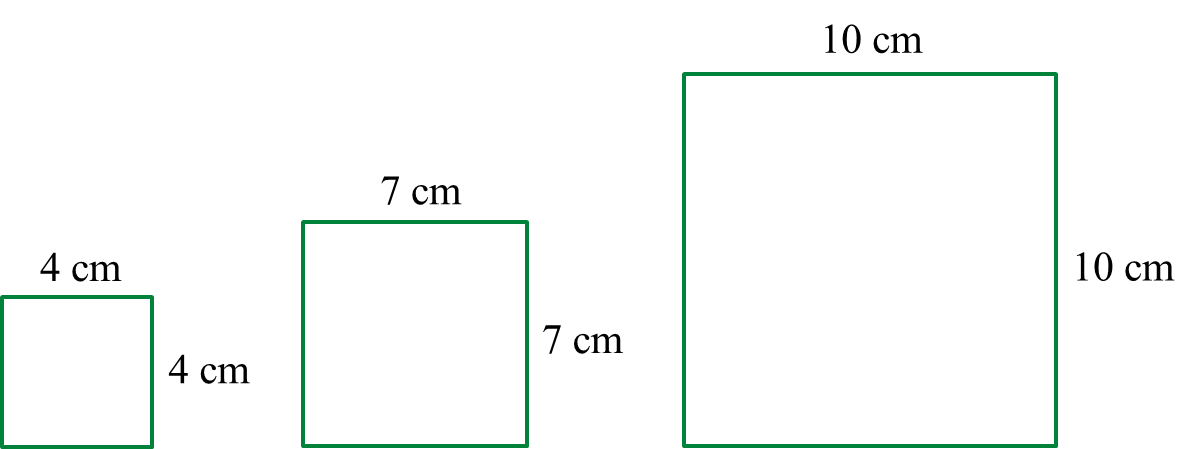# SPM Additional Mathematics 2018, Paper 1 (Question 14 – 16)

Question 14 (4 marks):
It is given that p, 2 and q are the first three terms of a geometric progression.
Express in terms of q
(a) the first term and the common ratio of the progression.
(b) the sum to infinity of the progression.

Solution:
(a)

(b)

Question 15 (3 marks):
A student has a wire with the length of 13.16 m. The student divided the wire into several pieces. Each piece is to form a square. Diagram 7 shows the first three squares formed by the student.Diagram 7

How many squares can be formed by the student?

Solution:

Question 16 (2 marks):
Given 2p + 2p = 2k. Express p in terms of k.

Solution:
$\begin{array}{l}{2}^{p}+{2}^{p}={2}^{k}\\ 2\left({2}^{p}\right)={2}^{k}\\ {2}^{p}=\frac{{2}^{k}}{{2}^{1}}\\ {2}^{p}={2}^{k-1}\\ p=k-1\end{array}$